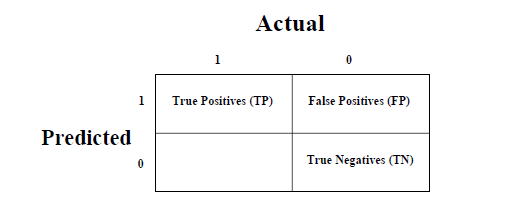# Machine Learning - Confusion Matrix

It is the easiest way to measure the performance of a classification problem where the output can be of two or more type of classes. A confusion matrix is nothing but a table with two dimensions viz. “Actual” and “Predicted” and furthermore, both the dimensions have “True Positives (TP)”, “True Negatives (TN)”, “False Positives (FP)”, “False Negatives (FN)” as shown below −The explanation of the terms associated with confusion matrix are as follows −

• True Positives (TP) − It is the case when both actual class & predicted class of data point is 1.

• True Negatives (TN) − It is the case when both actual class & predicted class of data point is 0.

• False Positives (FP) − It is the case when actual class of data point is 0 & predicted class of data point is 1.

• False Negatives (FN) − It is the case when actual class of data point is 1 & predicted class of data point is 0.

We can find the confusion matrix with the help of confusion_matrix() function of sklearn. With the help of the following script, we can find the confusion matrix of above built binary classifier −

from sklearn.metrics import confusion_matrix


Output

[[ 73 7]
[ 4 144]]


## Accuracy

It may be defined as the number of correct predictions made by our ML model. We can easily calculate it by confusion matrix with the help of following formula −

$$Accuracy = \frac{TP+TN}{TP+FP+FN+TN}$$

For above built binary classifier, TP + TN = 73+144 = 217 and TP+FP+FN+TN = 73+7+4+144=228.

Hence, Accuracy = 217/228 = 0.951754385965 which is same as we have calculated after creating our binary classifier.

## Precision

Precision, used in document retrievals, may be defined as the number of correct documents returned by our ML model. We can easily calculate it by confusion matrix with the help of following formula −

$$Precision = \frac{TP}{TP+FP}$$

For the above built binary classifier, TP = 73 and TP+FP = 73+7 = 80.

Hence, Precision = 73/80 = 0.915

## Recall or Sensitivity

Recall may be defined as the number of positives returned by our ML model. We can easily calculate it by confusion matrix with the help of following formula −

$$Recall = \frac{TP}{TP+FN}$$

For above built binary classifier, TP = 73 and TP+FN = 73+4 = 77.

Hence, Precision = 73/77 = 0.94805

## Specificity

Specificity, in contrast to recall, may be defined as the number of negatives returned by our ML model. We can easily calculate it by confusion matrix with the help of following formula −

$$Specificity = \frac{TN}{TN+FP}$$

For the above built binary classifier, TN = 144 and TN+FP = 144+7 = 151.

Hence, Precision = 144/151 = 0.95364

machine_learning_with_python_classification_algorithms_introduction.htm